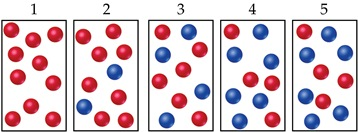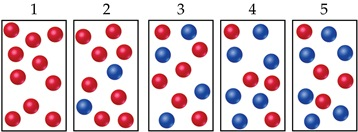# Problem: The following diagrams represent a hypothetical reaction A , with A represented by red spheres and B represented by blue spheres. The sequence from left to right represents the system as time passes.In which diagram(s) is the system in equilibrium?

###### FREE Expert Solution

We're being asked to determine which diagram(s) below is the system in equilibrium.A reaction reaches equilibrium:

• once the rate of the forward reaction equals the rate of the reverse reaction
• once at equilibrium there is no net change in the concentration of reactants or products

The sequence from left to right represents the system as time passes. Let's analyze each diagram:

83% (356 ratings)###### Problem Details

The following diagrams represent a hypothetical reaction A , with A represented by red spheres and B represented by blue spheres. The sequence from left to right represents the system as time passes.In which diagram(s) is the system in equilibrium?